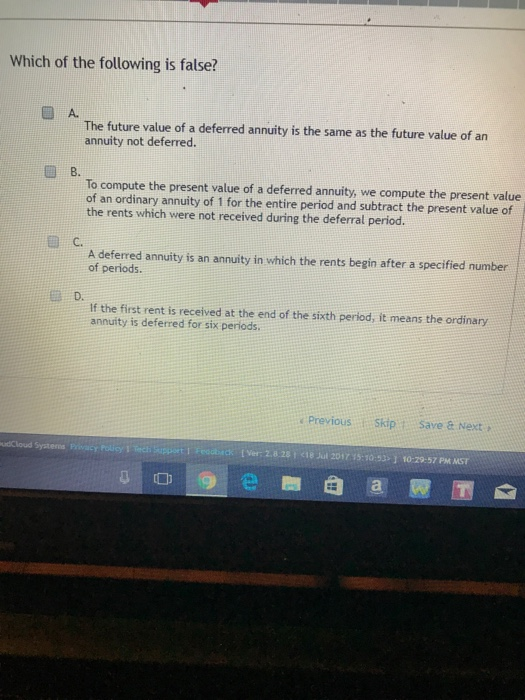# Question & Answer: Which of the following is false? A. The future value of a deferred annuity is the same as the future value of an annuity not deferred. B. To compu…..Which of the following is false? A. The future value of a deferred annuity is the same as the future value of an annuity not deferred. B. To compute the present value of a deferred annuity, we compute the present value of an ordinary annuity of 1 for the entire period and subtract the present value of the rents which were not received during the deferral period. C. A deferred annuity is an annuity in which the rents begin after a specified number of periods. D. If the first rent is received at the end of the sixth period, it means the ordinary annuity is deferred for six periods.